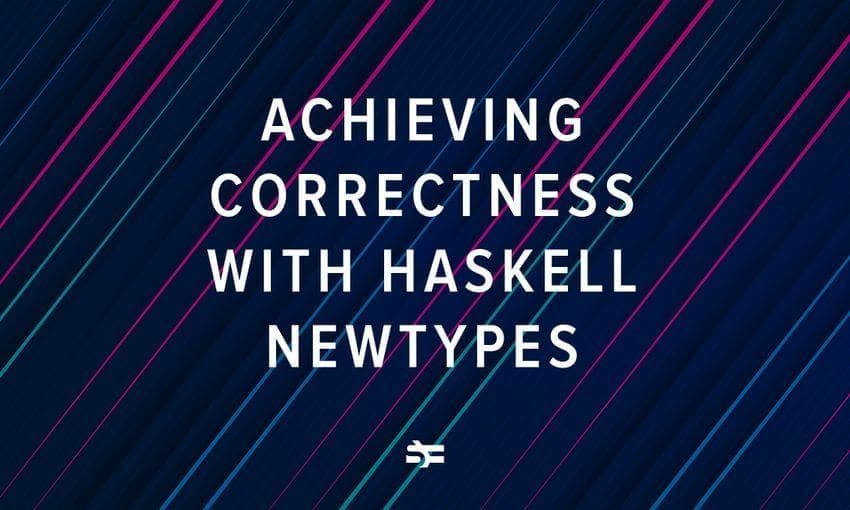This article is based on my talk with the same title, which I gave at the Haskell Love conference in 2020, where I give a cursory overview of GHC’s front-end pipeline, the internal Core language, and the desugaring pass.

Haskell is an expressive language with many features. On the one hand, it makes Haskell convenient, equipping the programmer with a rich arsenal of tools to get the job done and write high-quality software. On the other hand, developing a thorough understanding of these tools, of all the language features, takes time, effort, and experience.

One way to build an intuition for a language feature is to use it. Practice makes perfect, and by trial and error, you can discover the ins and outs of using certain parts of the language. However, the knowledge acquired this way may be superficial, and the mental model will only be as good as it was needed for the tasks at hand.

Deeper insight comes from a different perspective: you need to decompose a concept into its basic constituents. For example, what are multi-argument functions? In a curried language, we know that \a b c -> ... is much the same as \a -> \b -> \c -> .... Thus we have reduced the concept of a multi-argument function into a simpler concept of a single-argument function.

For a more involved example: what is do-notation? To grasp it, you need to think about how it is desugared into >>= and >> (and also <*> with -XApplicativeDo).

What about infix operators, if-then-else expressions, list comprehensions, type classes, type families, GADTs? How much Haskell is essential, and how much is sugar on top?

This becomes clear if we start thinking about the way Haskell programs are desugared into GHC’s Core: a small, elegant language, used as an intermediate representation in GHC’s compilation pipeline. The many features of Haskell are reducible to the few constructs of Core.

## The Essence of Desugaring

Desugaring translates a program that uses many different language constructs into a program that uses only a few.

For example, consider this snippet:

product [a + b, c + d]


1. Lists literals: [a, b, c, ...]
2. Operator application: x # y
3. Function application: f x

But we can rewrite it in such a way that it uses only function application:

product (
(:) ((+) a b) (
(:) ((+) c d) (
[])))


Granted, the end result is not as readable. But the building blocks used to write this program are simpler, and that’s the important bit.

## The Context of Desugaring

Desugaring is not just an abstract idea: it’s a concrete step of GHC’s pipeline. Every Haskell program is desugared into Core during compilation. So, to see the full picture, it’s helpful to consider the steps that occur prior to desugaring.

The input to the compiler is a string, a sequence of characters: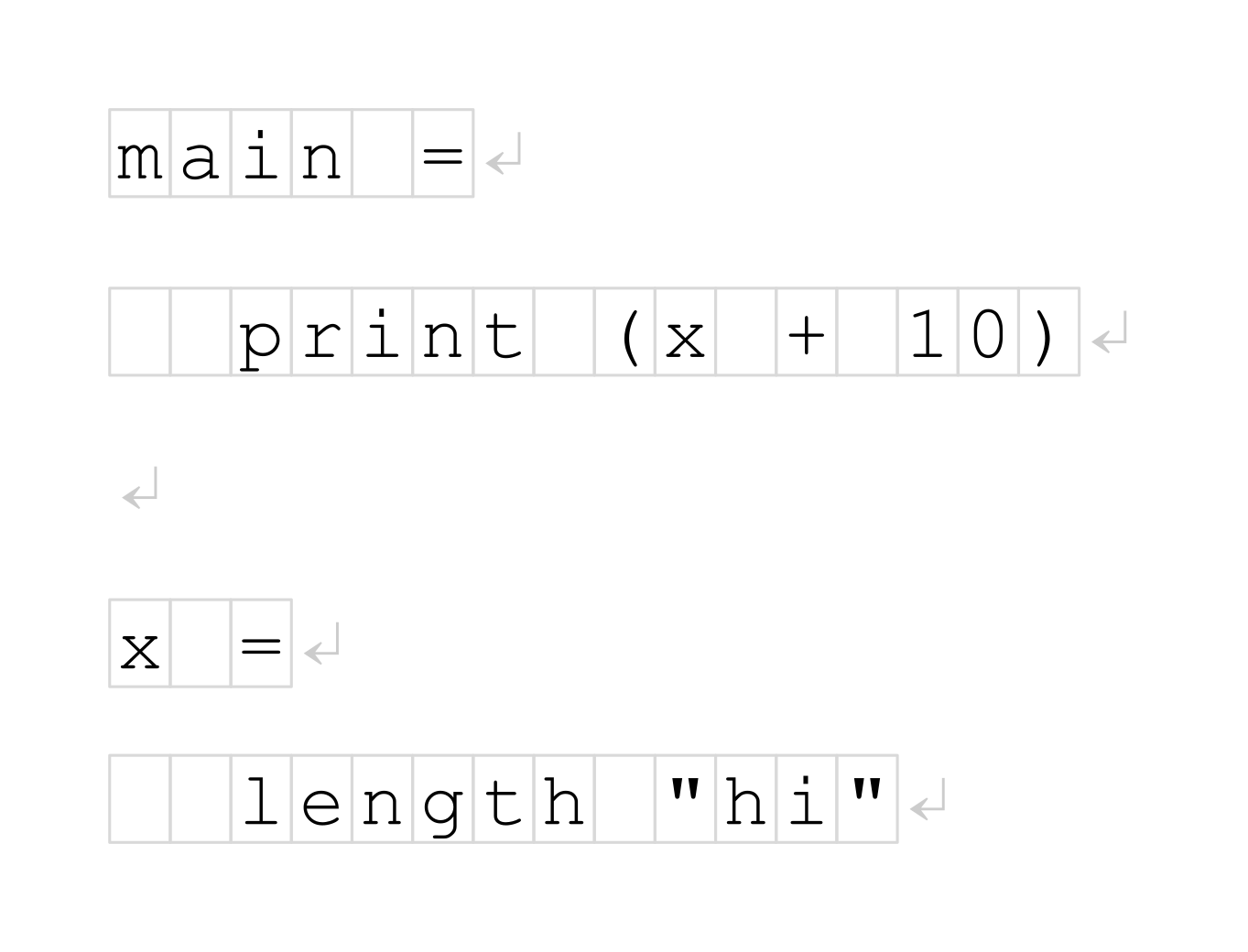Where would one begin to process this sequence? Actually, this is fairly well known. The first step is lexical analysis, which groups subsequences of these characters into labeled tokens: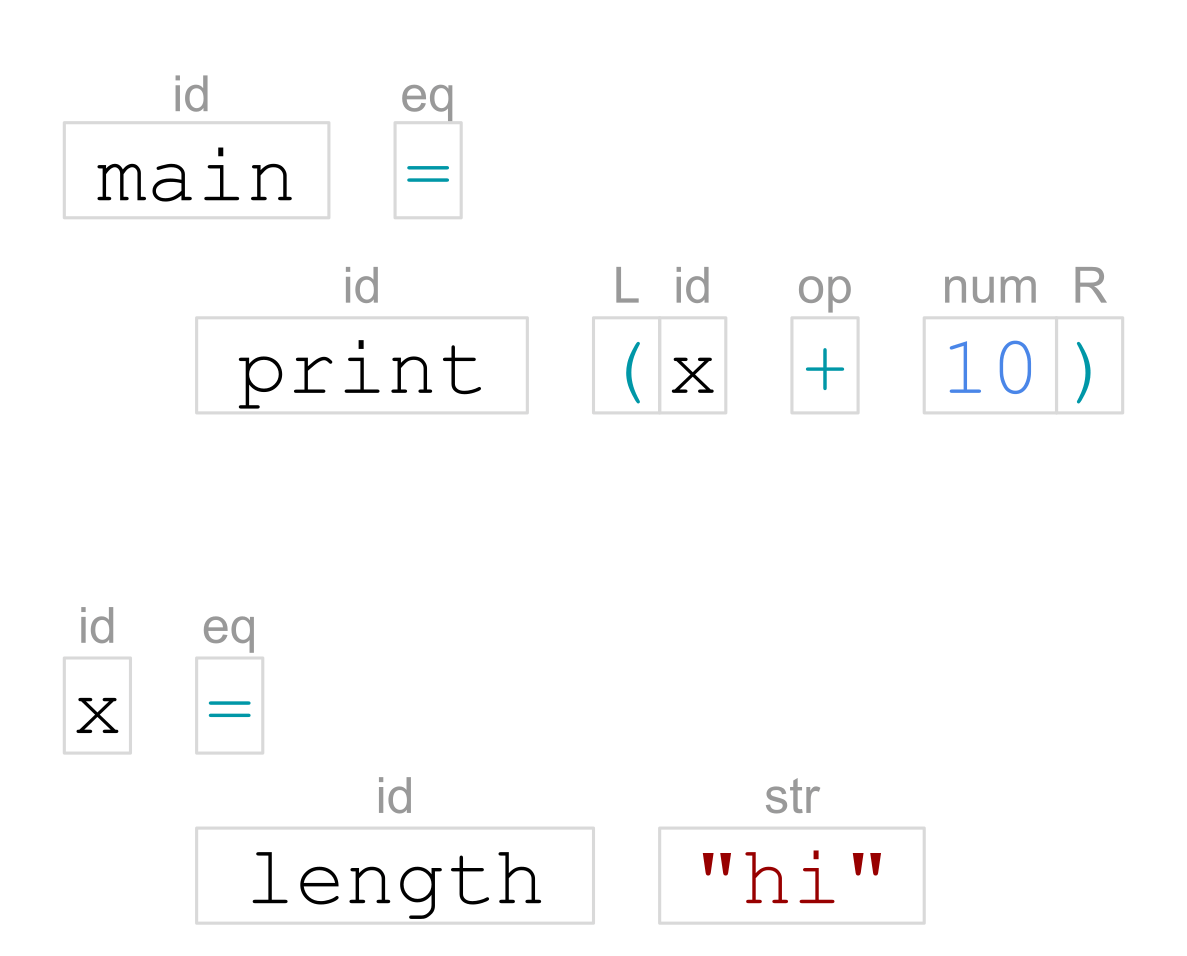Then the tokens are organized into a tree. That’s syntactic analysis: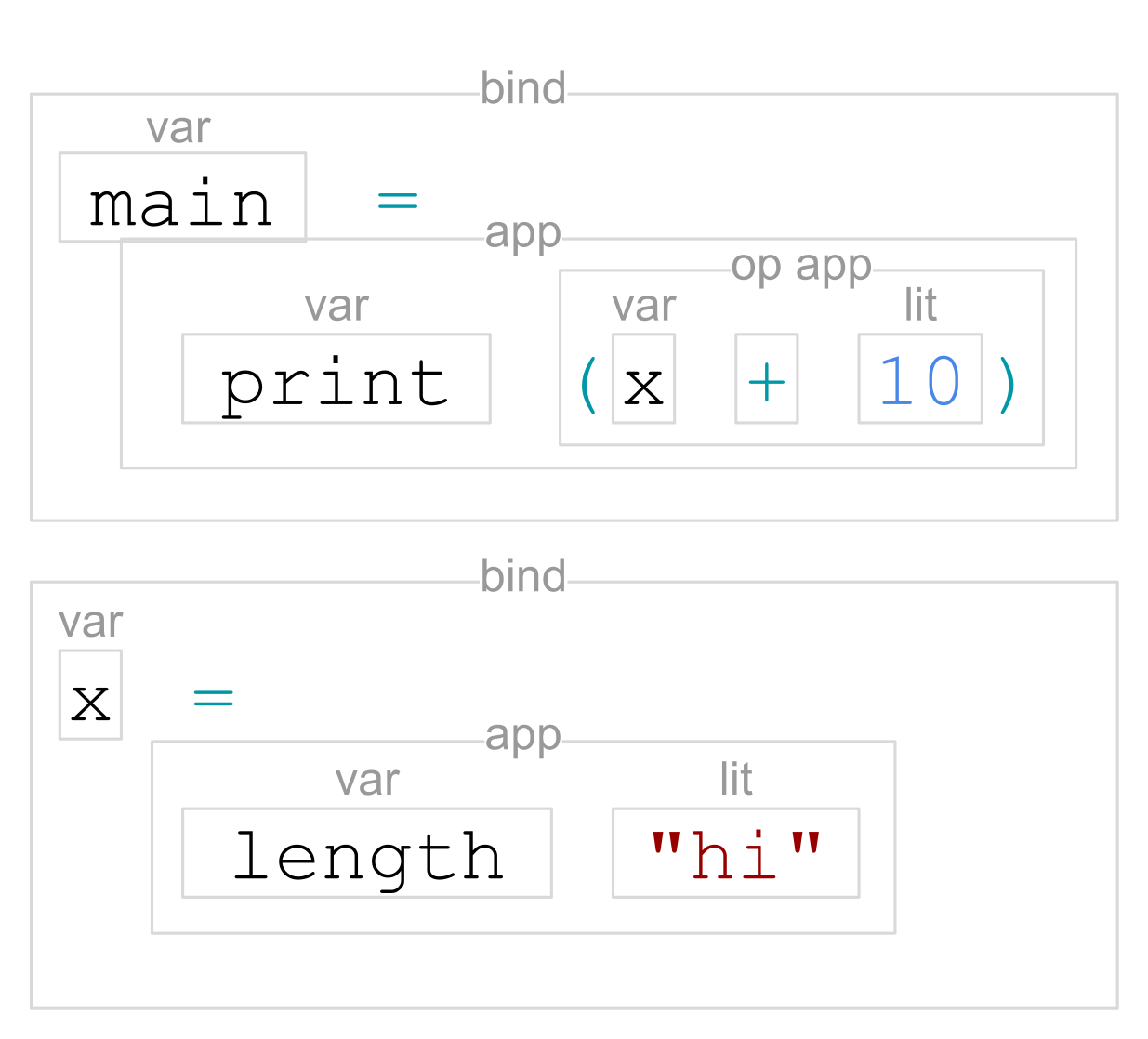The structure of this tree depends on the language we’re working with. In Haskell, a module contains declarations, such as data declarations, class declarations, function/variable definitions, and so on. In this example, we have two value bindings, marked as ‘bind’.

In a ‘bind’, there’s a pattern on the left-hand side and an expression on the right-hand side. In this example, the patterns are simply variable names, but we could also have as-patterns, view-patterns, matching on specific data constructors, and so on.

An expression can be one of many forms, but here we have:

• ‘app’ – function application, consisting of a function and its argument;
• ‘op app’ – operator application, consisting of two operands and an operator;
• ‘var’ – references to other named values;
• ‘lit’ – numeric and string literals.

Then we do name resolution, to figure out which name refers to what: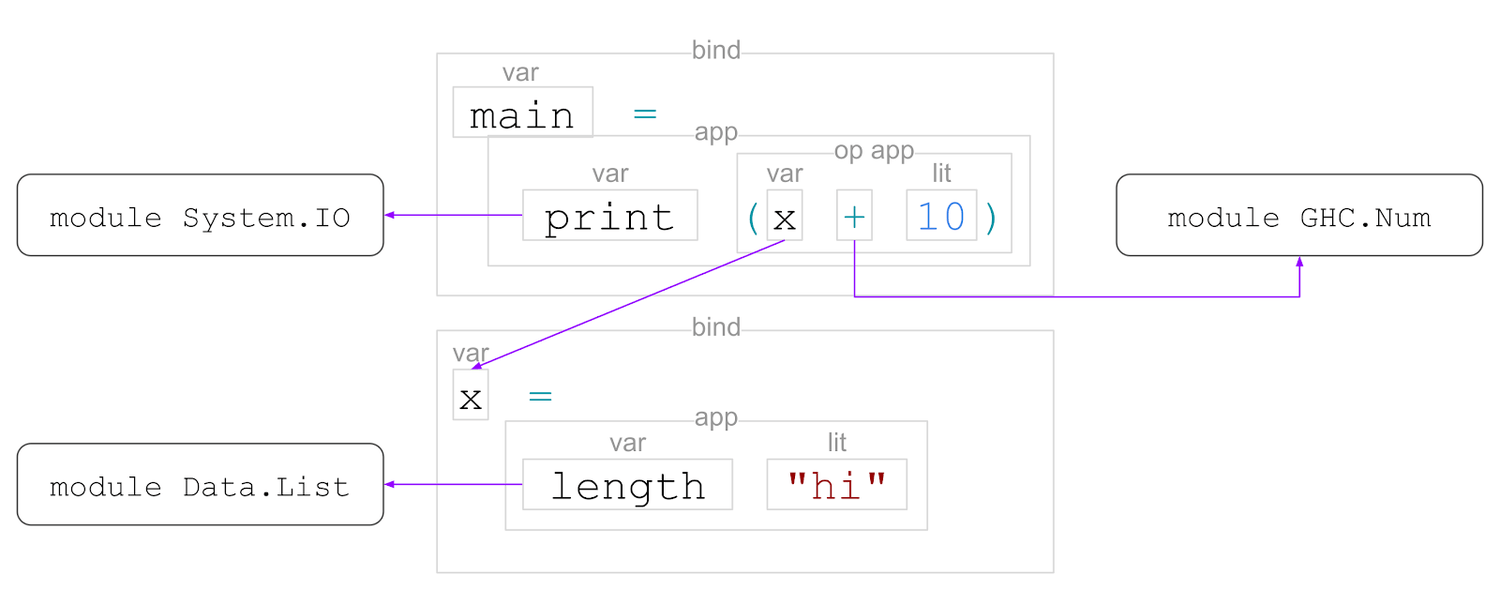Here, ‘print’, ‘+’, and ‘length’ are imported from other modules, whereas ‘x’ is defined in the same module.

And then, we analyse the program to check and infer the types of its expressions and subexpressions:

main :: IO ()
x :: Int


So that’s the GHC pipeline, or at least its front-end: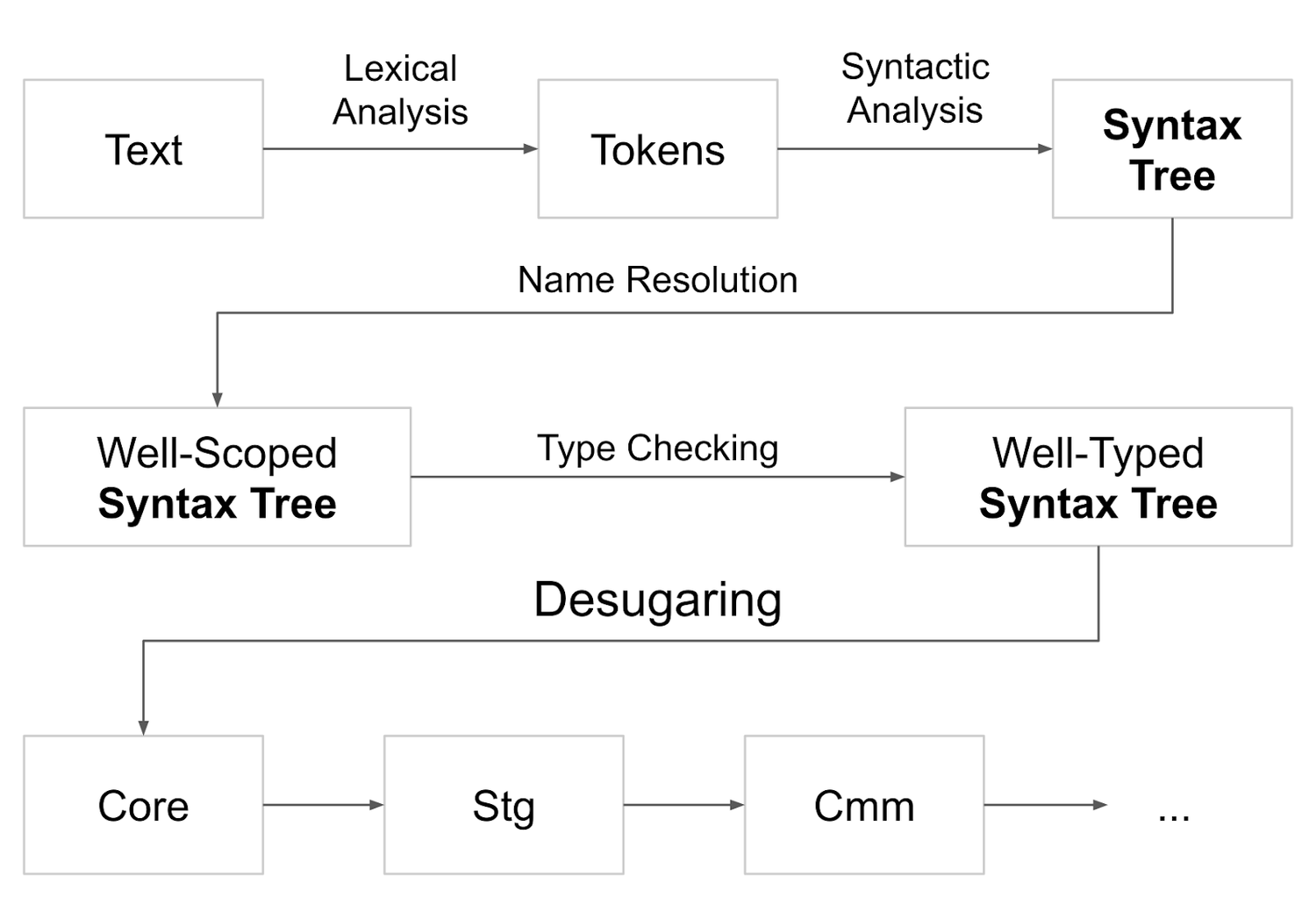• text to tokens;
• tokens to a syntax tree;
• add scoping information to the syntax tree;
• add type information to the syntax tree;

This leaves us with a well-scoped, well-typed syntax tree. This is the input to desugaring. And the output is a Core program, where Core is a language like Haskell, but it’s much smaller and with fewer features.

## The Simplicity of Core

To understand how Core is simpler, let’s first take a deeper look at Haskell. To represent a Haskell expression, GHC defines a type called HsExpr. If you open compiler/GHC/Hs/Expr.hs in GHC sources, you will see it:

data HsExpr p
= HsVar ...         --   v
| HsLit ...         --   "hello"
| HsApp ...         --   f x
| OpApp ...         --   x # y
| ...


Remember how syntactic analysis creates a node for each subexpression? And these nodes can be of different varieties, such as function application, operator application, variables, literals, etc.? HsExpr has a constructor for each node type: HsVar, HsLit, HsApp, OpApp, and so on.

And there are lots and lots of node types:

data HsExpr p
= HsVar ...         --   v
| HsLit ...         --   "hello"
| HsApp ...         --   f x
| OpApp ...         --   x # y
| HsAppType ...     --   f @t
| HsLam ...         --   \a b c -> d
| HsLet ...         --   let { v1 = e1; ... } in b
| ExprWithTySig ... --   e :: t
| ExplicitList ...  --   [a, b, c, ...]
| SectionL ...      --   (x #)
| SectionR ...      --   (# y)
| ExplicitTuple ... --   (a, b, c)
| HsCase ...        --   case e of { p1 -> e1; ... }
| HsLamCase ...     --   \case { p1 -> e1; ... }
| HsIf ...          --   if c then a else b
| HsMultiIf ...     --   if { | c1 -> a1 | ... }
| HsDo ...          --   do { v1 <- e1; e2; ... }
| RecordCon ...     --   MkR { a1 = e1; ... }
| RecordUpd ...     --   myR { a1 = e1; ... }
| ArithSeq ...      --   [a, b .. z]
| HsPar ...         --   (expr)
| NegApp ...        --   -x
| HsBracket ...     --   [| ... |]
| HsSpliceE ...     --   $( ... ) | HsProc ... -- proc v -> do { a1 <- e1 -< v1; ... } | HsStatic ... -- static e | HsOverLabel ... -- #lbl | ...  And that’s just expressions. There are also patterns, defined in compiler/GHC/Hs/Pat.hs: data Pat p = WildPat ... -- _ | VarPat ... -- v | LazyPat ... -- ~p | BangPat ... -- !p | AsPat ... -- x@p | ParPat ... -- (p) | ListPat ... -- [a, b, c, ...] | TuplePat ... -- (a, b, c, ...) | ConPat ... -- MkT p1 p2 p3 ... | ViewPat ... -- (f -> p) | LitPat ... -- "hello" | SigPat ... -- p :: t | NPat ... -- 42 | NPlusKPat ... -- n+42 | SplicePat ... --$( ... )
| ...


And types, defined in compiler/GHC/Hs/Type.hs:

data HsType p
= HsForAllTy ...    --   forall a b c. t
| HsQualTy ...      --   ctx => t
| HsTyVar ...       --   v
| HsAppTy ...       --   t1 t2
| HsAppKindTy ...   --   t1 @k1
| HsFunTy ...       --   t1 -> t2
| HsListTy ...      --   [t]
| HsTupleTy ...     --   (a, b, c, ...)
| HsOpTy ...        --   t1 # t2
| HsParTy ...       --   (t)
| HsIParamTy ...    --   ?x :: t
| HsStarTy ...      --   *
| HsKindSig ...     --   t :: k
| HsSpliceTy ...    --   $( ... ) | HsTyLit ... -- "hello" | HsWildCardTy ... -- _ | ...  In compiler/GHC/Hs/Decls.hs there are data declarations, classes, type families, instances, and so on: data TyClDecl p = FamDecl ... -- type family T | SynDecl ... -- type T = ... | DataDecl ... -- data T = ... | ClassDecl ... -- class C t where ... data InstDecl p = ClsInstD ... -- instance C T where ... | DataFamInstD ... -- data instance D T = ... | TyFamInstD ... -- type instance F T = ...  That’s not all, of course. You can browse compiler/GHC/Hs/... to see more. What about Core? Here’s the entirety of its syntax: data Expr = Var Id | Lit Literal | App Expr Expr | Lam Var Expr | Let Bind Expr | Case Expr Var Type [Alt] | Cast Expr Coercion | Type Type | Coercion Coercion | Tick ... -- unimportant type Alt = (AltCon, [Var], Expr) data AltCon = DataAlt DataCon | LitAlt Literal | DEFAULT data Bind = NonRec Var Expr | Rec [(Var, Expr)] data Type = TyVarTy Var | AppTy Type Type | TyConApp TyCon [Type] | ForAllTy TyCoVarBinder Type | FunTy Mult Type Type | LitTy TyLit | CastTy Type Coercion | CoercionTy Coercion  Its expression syntax has only nine constructs: • variables (Var) • literals (Lit) • function application (App) • lambdas (Lam) • let-bindings (Let) • case-expressions (Case) • casts (Cast) • coercions (Coercion) If you learn what these are, you know Core. And if you know both Core and how Haskell programs are desugared into it, then you can easily reason about the menagerie of Haskell language features. At a first approximation, you can think of Core as a subset of Haskell plus coercions (and casts, these two are closely related). That’s not the full story, though. For example, there are also differences in strictness, as case in Core is always strict. Here are some resources if you want to delve deeper into this: However, as a starting point, it’s sufficient to assume that Core is a subset of Haskell. ## Desugaring by Example Now let’s see how Haskell programs are transformed into Core by looking at specific examples. We’ll start with the most basic features and progress to more complex ones. ### Infix Operators Infix operators are translated into function applications: Haskell Core a && b  (&&) a b  There isn’t much to it. But keep in mind that in Core, all variable occurrences have type information, so a more accurate translation would look like this: ((&&) :: Bool -> Bool -> Bool) (a :: Bool) (b :: Bool)  However, more often than not, I will omit type annotations to save visual space. ### Function Bindings Bindings in Core always have a single variable name on the left-hand side. Function bindings are desugared into lambdas: Haskell Core f :: Integer -> Integer f x = x  f = \ (x :: Integer) -> (x :: Integer)  Also, there are no separate type signatures. All type information is stored inline. ### Multi-Argument Function Bindings Multi-argument functions are translated into nested lambdas. In Core, all lambdas are single-argument: Haskell Core f x y = not x && y  f = \(x :: Bool) -> \(y :: Bool) -> (&&) (not x) y  This is also true for hand-written multi-argument lambdas: Haskell Core f = \x y -> not x && y  f = \(x :: Bool) -> \(y :: Bool) -> (&&) (not x) y  This treatment of multi-argument functions may be familiar to you if you’ve heard of currying. ### Pattern Bindings Pattern bindings are desugared into several Core bindings: one for the entire value, and additional bindings for each of its parts. Haskell Core a :: Integer b :: Bool (a, b) = (1, True)  ab = (1, True) a = case ab of (,) x y -> x b = case ab of (,) x y -> y  The parts are extracted using case-expressions. ### Operator Sections Operator sections are desugared into lambdas: Haskell Core (a &&)  \b -> (&&) a b  (&& b)  \a -> (&&) a b  However, with the -XPostfixOperators extension, left sections are η-reduced, so (a &&) is desugared into (&&) a instead. Tuple sections are translated into lambdas, too: Haskell Core (,True,)  \a -> \b -> (,,) a True b  ### Multi-Argument Pattern Matching Functions that match on several arguments are translated into nested case-expressions: Haskell Core and True True = True and True False = False and False True = False and False False = False  and = \(a :: Bool) -> \(b :: Bool) -> case a of False -> case b of False -> False True -> False True -> case b of False -> False True -> True  Matching with ‘case’ in Core is not as sophisticated as in surface Haskell. It forces the argument to WHNF, and then works more like a ‘switch’ statement in C, comparing constructor tags. So to match on multiple variables, we need multiple case-expressions. ### Deep Pattern Matching A pattern match that requires looking deep into the data will desugar to nested case-expressions, too: Haskell Core f (Left (Just "")) = True f (Right ()) = True f _ = False  f = \e -> case e of Left l -> case l of Nothing -> False Just s -> case s of [] -> True (:) a b -> False Right r -> case r of () -> True  In the first function clause, we check the input value in steps. We check that we are given: 1. The Left constructor of Either, which contains… 2. The Just constructor of Maybe, which contains… 3. An empty string, represented by the [] constructor of built-in lists. In Core, we have a case-expression for every such step. ### Lambda Case The -XLambdaCase extension… is pretty self-explanatory. Haskell Core \case True -> False False -> True  \x -> case x of True -> False False -> True  ### If-Then-Else An if-then-else expression is translated into a simple case-expression: Haskell Core if c then a else b  case c of False -> b True -> a  The translation is quite direct and makes one question why if-then-else is in the language in the first place. But things get a bit more interesting with -XMultiWayIf, where we start seeing nested case-expressions again: Haskell Core if | c1 -> a1 | c2 -> a2 | c3 -> a3 | otherwise -> a4  case c1 of False -> case c2 of False -> case c3 of False -> a4 True -> a3 True -> a2 True -> a1  ### The seq Function The ‘seq’ function, which forces evaluation of its argument to weak-head normal form, is desugared into a case-expression, relying on the fact that in Core, case-expressions are strict: Haskell Core seq a b  case a of _ -> b  Bang patterns are virtually the same thing as ‘seq’, as they are also translated into strict case-expressions: Haskell Core f, g :: Bool -> Bool f x = x g !x = x  f = \x -> x g = \x -> case x of _ -> x  Here, f is the identity function, whereas g also forces its argument to WHNF. ### Parametric Polymorphism Now, for something more interesting, let’s talk about parametric polymorphism. That’s finally when we start seeing parts of Core that are not easily observed in Haskell. In Core, whenever you have a type parameter, it must be bound by a lambda, as if it was a normal function parameter: Haskell Core id :: forall a. a -> a id x = x  id = \ @(a :: Type) -> \ (x :: a) -> x  Here, in the id function, we have a lambda for the a type parameter, and then a lambda for the x value parameter (of type a). When we use a polymorphic function, such as id, in surface Haskell we just give it the value arguments. But in Core, we must also supply the type arguments: Haskell Core t :: Bool t = id True  t = id @Bool True  This gives a useful perspective on the -XTypeApplications extension. It is, in fact, a part of Core making an appearance in surface Haskell, except in Core, it’s compulsory. As another example, when you construct a tuple of three elements, you’d pass six arguments in Core. First, the elements types, and then the elements: Haskell Core p = (True, 'x', "Hello")  p = (,,) @Bool @Char @String True 'x' "Hello"  ### Existential Quantification Why is passing type parameters explicitly a valuable idea? One reason is that it demystifies existential quantification, with which I, personally, struggled for a long time. Looking at Core reveals that existential type parameters behave just like other fields of a data constructor: Haskell Core data E = forall a. MkE [a] lenE :: E -> Int lenE (MkE xs) = GHC.List.length xs  lenE = \(e :: E) -> case e of MkE @a xs -> GHC.List.length @a xs  When we match on MkE here, in the original program only xs is brought into scope. But in the Core program, the existential type variable is also brought into scope, and then passed to the length function. There’s also a guarantee that type information will be erased in a later pass, so there’s no overhead at runtime. But at the Core level, it really behaves as if it’s stored alongside other data. ### Classes and Dictionary Passing In Core, there are no type classes. Class instances are passed around in Core explicitly, as ordinary data. These values are called “dictionaries”, but there isn’t that much special about them. Unlike type parameters, they are passed at runtime: Haskell Core f :: Num a => a -> a f x = x + x  f = \ @(a :: Type) -> \ ($dNum :: Num a) ->
\ (x :: a) ->
(+) @a $dNum x x  Here, we have a Num a constraint, but in Core, it’s just another function parameter, which we bind to the $dNum variable. This $dNum value contains the implementations of (+), (*), and other Num methods for the given choice of a. So, while in surface Haskell it might appear that f has one parameter x, after desugaring to Core it actually has three parameters: 1. a :: Type, the type of its input/output (for example, Int or Double) 2. $dNum :: Num a, the class dictionary with method implementations
3. x :: a, the input value

And when you use a polymorphic function with a class constraint, you need to pass the class dictionary to it:

f :: Int -> Int
f x = x + x

f =
\(x :: Int) ->
(+) @Int $dNumInt x x  The $dNumInt here contains the implementations of all Num methods for Int. It’s a value created by the Num Int instance:

instance Num Int where ...

$dNumInt = ...  As you can see, class instances are desugared into value bindings. ### Do-notation Let’s see how all of this plays out in do-notation. Every bind corresponds to a use of the (>>=) operator: Haskell Not quite Core f act = do x <- act y <- act return (x && y)  f = \act -> act >>= \x -> act >>= \y -> return (x && y)  But we know that there are no operators in Core. Also, (>>=) is polymorphic, so we’ll need to pass a type parameter and the Monad instance dictionary to it. So the actual Core looks like this: Haskell Core f act = do x <- act y <- act return (x && y)  f = \ @(m :: Type -> Type) -> \ ($dMonad :: Monad m) ->
\ (act :: m Bool) ->
(>>=)  @m $dMonad @Bool @Bool act (\x -> (>>=) @m$dMonad @Bool @Bool act (\y ->
return @m \$dMonad @Bool ((&&) x y)))

1. First, we take the m type variable as input. At use sites of f, the user will instantiate m to something like IO, Maybe, [], Either e, Reader r, Writer w, etc.
2. The second parameter is the dictionary of the Monad type class. It contains implementations of (>>=) and return for the given choice of m.
3. And then we have the monadic action itself, act.

The rest of the function is what one would expect: we call the (>>=) function to chain monadic actions, but we also supply the type parameters and the Monad class dictionary to it.

While monads may seem quite mysterious at first, involving type classes, parametric polymorphism, and the do-notation sugar on top of it all, they end up as a bunch of lambdas and function applications in the end.

### Coercions and Casts

There are two extra features of Core that are not present in Haskell – coercions and casts. These two are closely tied to each other.

data Expr
= Var   Id
| Lit   Literal
| App   Expr Expr
| Lam   Var Expr
| Let   Bind Expr
| Case  Expr Var Type [Alt]
| Cast  Expr Coercion
| Type  Type
| Coercion Coercion


One way to observe them in the generated Core is to use an equality constraint. Let’s start with the identity function, and spice things up by adding an equality constraint:

Bland Spicy
id :: a -> a
id x = x

id :: (a ~ b) => a -> b
id x = x



Unlike the familiar identity function which takes a to a, it takes a to b, but at the same time it requires a proof that a and b are the same type. When we translate this program into Core, where we do everything explicitly, there are two gaps to fill:

id :: (a ~ b) => a -> b
id x = x

id =
\ @(a :: Type) ->
\ @(b :: Type) ->
\ ???₁ ->
\ (e :: a) ->
e ???₂ :: b


Of course we bind the type variables, a and b, but then there ought to be some sort of binding for the equality constraint. That’s the first gap, and that’s where coercions come into play:

id :: (a ~ b) => a -> b
id x = x

id =
\ @(a :: Type) ->
\ @(b :: Type) ->
\ (co :: a ~ b) ->
\ (e :: a) ->
e ???₂ :: b


Coercions serve as evidence of type equality. If you’ve got a coercion (such as co above) of type a ~ b, then you can convert an expression of type a to an expression of type b (or vice versa, as equality is symmetric).

And that’s what we need to do to fill the second gap. The e value has type a, but the function must return a value of type b. We put the coercion to use by referencing it in a cast:

id :: (a ~ b) => a -> b
id x = x

id =
\ @(a :: Type) ->
\ @(b :: Type) ->
\ (co :: a ~ b) ->
\ (e :: a) ->
e |> co


We write the cast as |>, and its role is to guide Core’s type checker. Unlike Haskell’s type checker, it doesn’t do any sort of inference or advanced reasoning. Explicit coercions and casts make it simple and straightforward to type check programs.

An interesting thing about coercions is that you can put them in data constructors. We’ve already seen how storing type variables in data constructors explains existential quantification. Coercions in data constructors are the basis of GADTs:

data G a =
(a ~ Int) => MkG a

f :: G a -> Int
f (MkG n) = n

f = \ @(a :: Type) ->
\  (g :: G a) ->
case g of
MkG co n -> n |> co


Here MkG also stores a proof that a is equal to Int. So when we pattern match on MkG, in Core we get a coercion that serves as evidence of this equality. And then we can use it in a cast: we have n of some unknown type a, but we need to return an Int. The coercion co :: a ~ Int is exactly what we need to convince the type checker that this is, in fact, fine.

And when constructing a value of type G a, one must supply this coercion, so it’s not possible to get a non-divergent expression of type, say, G Bool, as Haskell’s type checker will not produce a coercion of type Bool ~ Int.

### Type Families

Coercions are also used to desugar type families. When you have a type instance, in Core it corresponds to an axiom, represented by a coercion:

type family F a
type instance F Bool = String

fBoolString =
Axiom :: F Bool ~ String


And then this coercion, created by a type family instance, can be used in casts.

### Proof Language

Coercions form the internal proof language of GHC: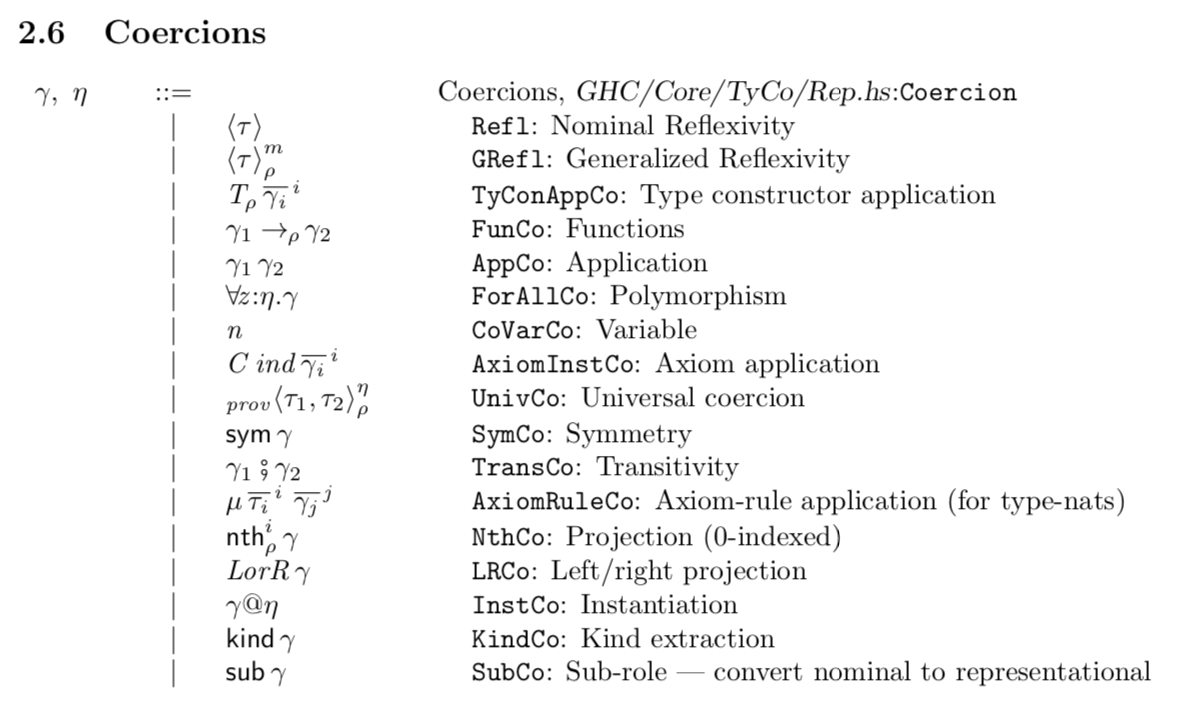But the specifics of this language are more of a technicality, and you don’t need them unless you’re working on the formalism or extending the GHC’s type checker.

The important bit is the idea of explicitly passing around equality proofs and using them in casts.

## Conclusion

The rich variety of Haskell features can be reduced to the few features of GHC’s Core. The compiler pass that performs this conversion is called desugaring, and it occurs after parsing, name resolution, and type checking.

Thinking about the way Haskell language constructs are desugared into Core provides a deeper understanding of these features, rather than a superficial familiarity. For example, it provides a clear intuition for existential quantification, GADTs, and do-notation.

In everyday programming, reading compiler-generated Core can be useful when you are investigating the performance of a particular piece of code. Core is also a valuable piece of knowledge if you ever plan to contribute to GHC or write a proposal.

If you are into papers, a good starting point to learn more about Core is “System F with Type Equality Coercions", and then “System FC with Explicit Kind Equality".

Let me know what you think about GHC’s Core and desugaring in the comments of Reddit!

Serokell’s team of Haskell experts specializes in developing cutting-edge software customized to the unique needs of modern high-tech businesses. Contact us today to discuss the details of your current or future project.More from Serokell